Subsections

# Limits of functions.

## Purpose

The purpose of this lab is to use Maple to become more familiar with limits of functions, including one-sided limits.

## Background

### Simple limits and Maple

Limits of many functions and expressions can be computed in Maple with the limit command. Some examples are given below.
  > limit(x^2+2*x,x=2);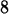> limit(sin(x)/x,x=0);> f := x -> (x+3)/(x^2+7*x+12) ;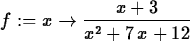> limit(f(x),x=-3);> limit(f(x),x=-4);If the limit exists, Maple can usually find it. In cases where the limit doesn't exist, Maple gives the answer infinity for an unbounded limit or gives a range like -1..1 if the limit doesn't exist, but the expression or function is bounded. See the examples below.
  > limit(1/x,x=0);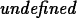> limit(sin(1/x),x=0);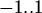You can also use Maple to compute limits as x goes to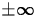as shown below.
  > f(x);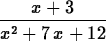> limit(f(x),x=infinity);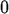> limit(f(x),x= -infinity);The formal definition for a limit is given below.

Definition 342

We say that the number L is the limit of f(x) as x approaches a provided that, given any number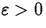, there exists a number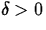such that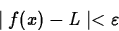for all x such that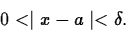This definition may seem complicated, but its graphical interpretation is not so bad. It says that if you plot f(x) - L with the y range set to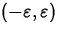you can always choose a value of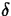small enough so that when you shrink the x plot range to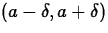and plot the function, its graph will not intersect the top or the bottom edges of your plot. For example, suppose f(x)=x2, a=2 and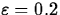. Then any value ofsmaller than about 0.049 will work. To see what is going on, look at the plots generated by the following commands.

  > f := x -> x^2;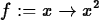> limit(f(x),x=2);> plot({-0.2,0.2,f(x)-4},x=2-0.1..2+0.1,y=-0.2..0.2);

  > plot({-0.2,0.2,f(x)-4},x=2-0.048..2+0.048,y=-0.2..0.2);

In the first of the two plot commands, the value ofis 0.1. This is too large, since the graph intersects the lines y=-0.2 and y=0.2. The value of 0.048 forin the second plot command, however, is small enough, since the graph of f(x) goes off the sides of the plot. Make sure that you understand this example. If you don't understand, ask for help.

### Limits of more complicated functions

It should be no secret by now that for most functions f(x) defined by a single formula,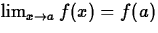when f(a) exists. For more complicated functions, this may not be true. For dealing with some of these exceptional cases, we need to define right-hand and left-hand limits. Loosely speaking, the right-hand limit of f(x) at a is L if f(x) approaches L as x approaches a from the right. That is, the values of x satisfy x > a. The left-hand limit is defined in an analogous manner, with the values of x approaching a from the left. Maple can compute these special limits with commands like those shown below. The Maple floor function is actually the greatest integer function.
  > plot(floor(x),x=0..4);

  > limit(floor(x),x=1,right);> limit(floor(x),x=1,left);The floor function is one of Maple's defined functions, so you might expect things to work properly. If you define your own function in a piecewise fashion, however, a different approach is needed. For example, suppose you wanted to find the limit as x approaches 2 for the following function: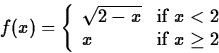You would need to execute the following commands:
  > f1 := x -> sqrt(2-x);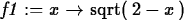> limit(f1(x),x=2,left);> f2 := x -> x;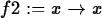> limit(f2(x),x=2,right);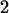From this, we see that the limit of f as x approaches 2 does not exist since the limit from the left does not equal the limit from the right.Next: About this document ... Up: Labs and Projects for Previous: Labs and Projects for

Christine Marie Bonini
1/27/1999